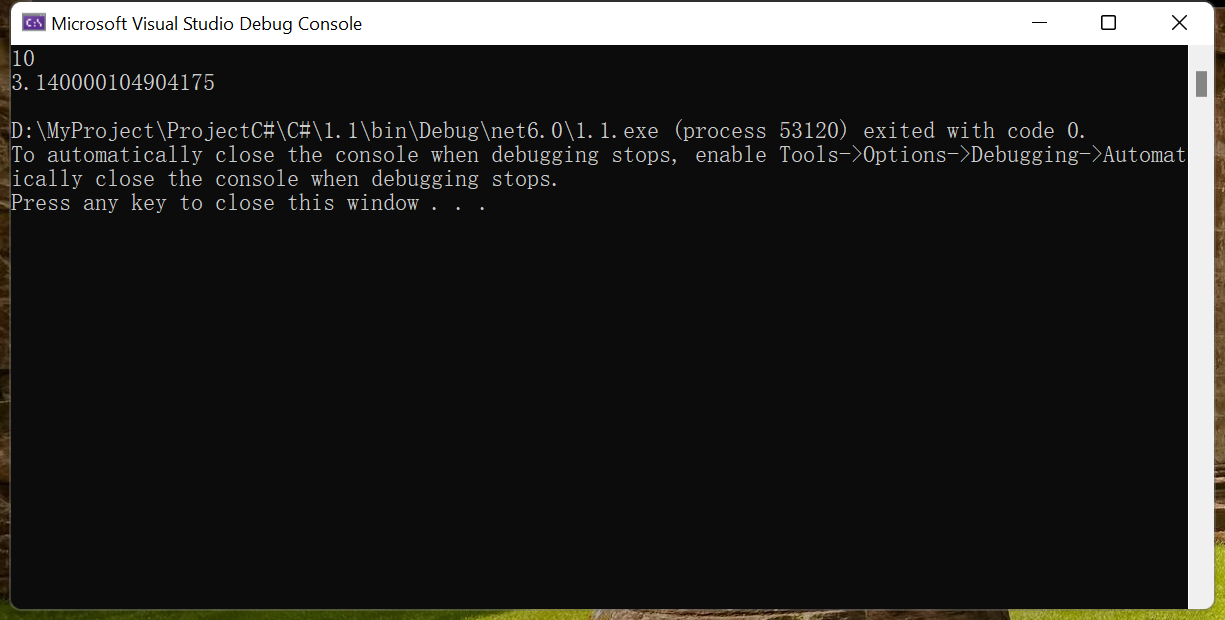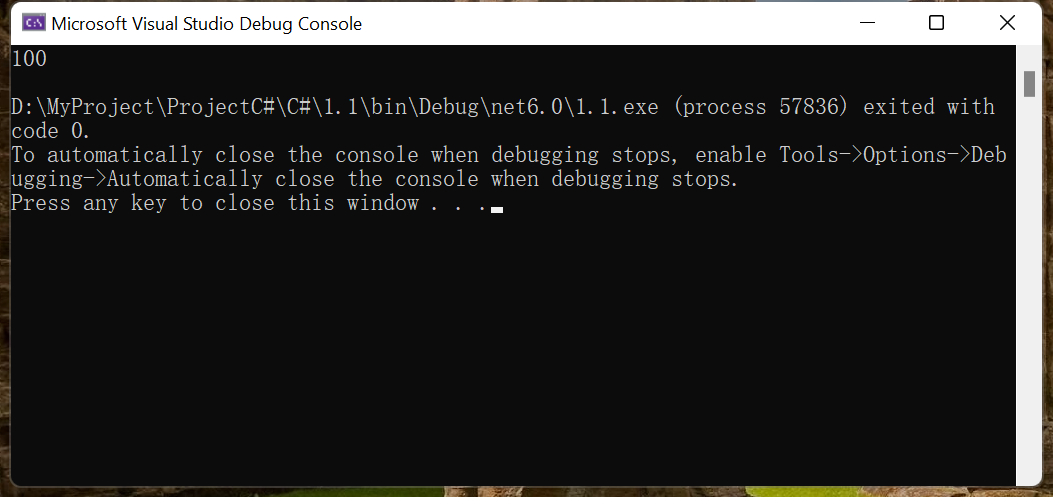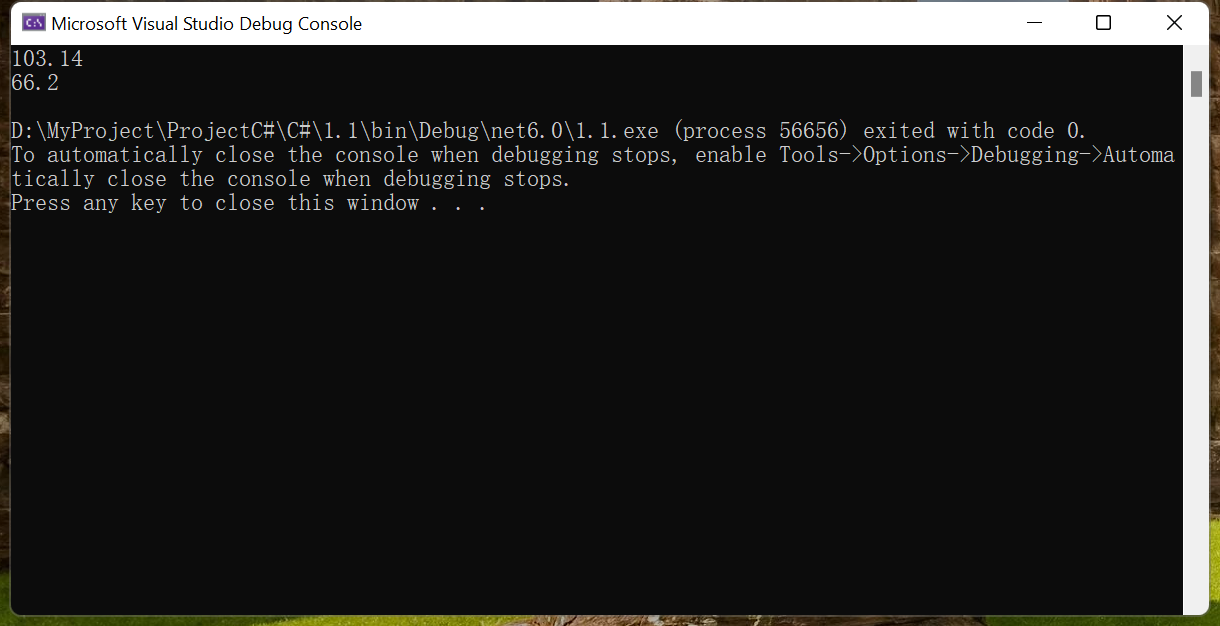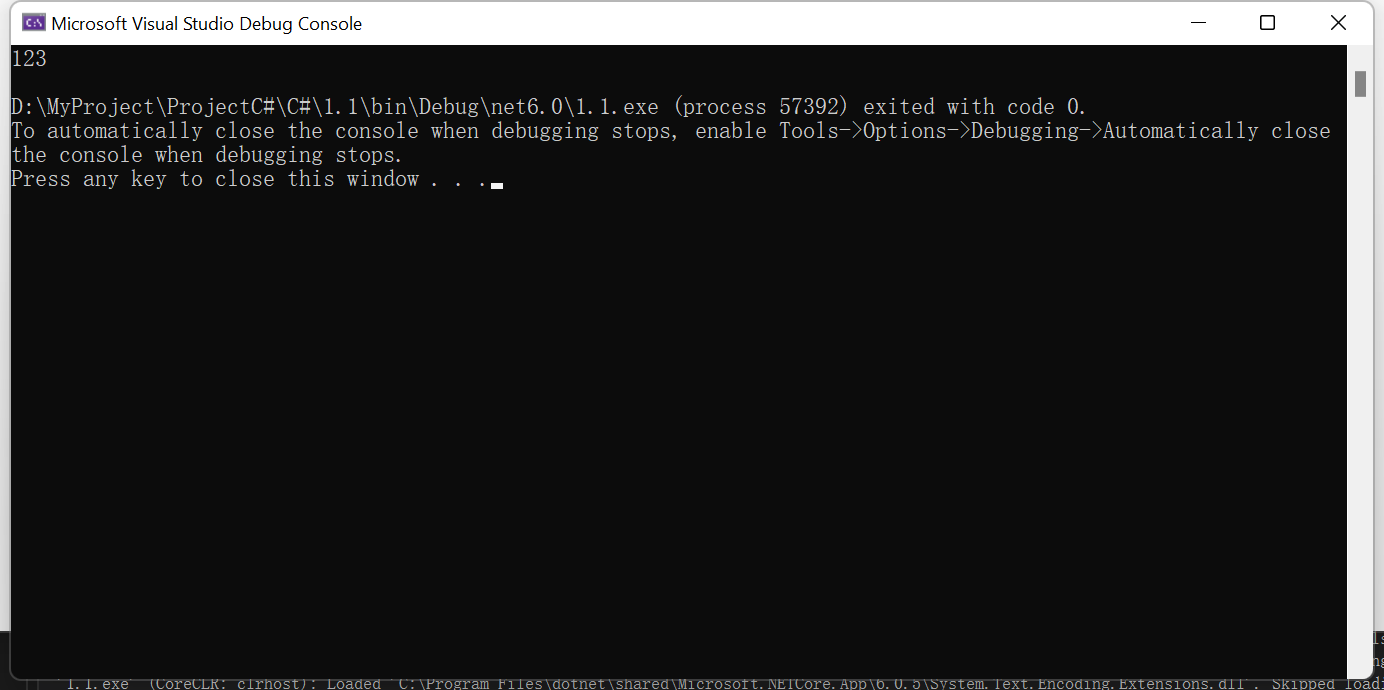ew的小天地 科技 12.C# 类型转换

# 12.C# 类型转换

• 隐式类型转换 这些转换是C#默认的以安全方式进行的转换，不会导致数据丢失。例如：从小的整数类型转换为大的整数类型，从派生类转换为基类。
• 显式类型转换 显式类型转换即强制类型转换。显式转换需要强制转换运算符，而且强制转换会造成数据丢失。

int a = 10;ndouble b = a;//将int隐式转换为double,从低到高nfloat f = 3.14f;nb = f;//将float转换为double,从低到高nfloat g = b;//这个就会出错了，double比float要高nConsole.WriteLine(a);nConsole.WriteLine(b);• 从 sbyte 类型到 short,int,long,float,double,或 decimal 类型。
• 从 byte 类型到 short,ushort,int,uint,long,ulong,float,double,或 decimal 类型。
• 从 short 类型到 int,long,float,double,或 decimal 类型。
• 从 ushort 类型到 int,uint,long,ulong,float,double,或 decimal 类型。
• 从 int 类型到 long,float,double,或 decimal 类型。
• 从 uint 类型到 long,ulong,float,double,或 decimal 类型。
• 从 long 类型到 float,double,或 decimal 类型。
• 从 ulong 类型到 float,double,或 decimal 类型。
• 从 char 类型到 ushort,int,uint,long,ulong,float,double,或 decimal 类型。
• 从 float 类型到 double 类型。

byte b = 10;nint i = b;nConsole.WriteLine(i);nnuint x = 100;nlong y = x;//这里要是将uint转int也会出错，转long没有出错nConsole.WriteLine(y);int x = 100;ndouble y = 3.14;ndouble z = x + y;nConsole.WriteLine(z);nnchar a = 'A';//65ndouble d = 1.2;nConsole.WriteLine(a+d);double x = 4.2425;nint y = (int)x;nConsole.WriteLine(y);

Parse方法

Parse方法用于将字符串类型转换成任意基本类型，基本所有数据类都有这个方法

string x = "123";nConsole.WriteLine(int.Parse(x));nnstring y = "10.8";nConsole.WriteLine(double.Parse(y));

TryParse方法

string x = "123";nnint y = 0;nbool ret = int.TryParse(x, out y);//如果转换出错，返回false,如果正确，y就等于转换后的值

Convert方法

Convert方法是数据类型转换中最灵活的方法，它能够将任意数据类型的值转换成任意数据类型，前提是不要超出指定数据类型的范围。

Convert.ToInt16()

Convert.ToInt32()

Convert.ToInt64()

Convert.ToChar()

Convert.ToString()

Convert.ToDateTime()

Convert.ToDouble()

Conert.ToSingle()

string x = "123";nint y=Convert.ToInt32(x);nConsole.WriteLine(y);